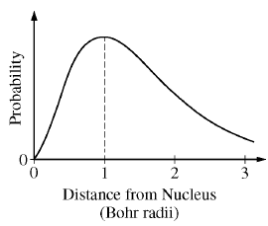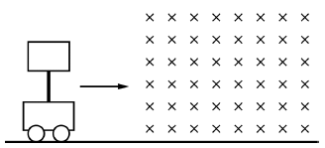# 2017 AP Physics 2物理Algebra-Based真题系列之Practice Exam选择题免费下载

### 真题与答案下载### 真题下载请前往【纯真题】小程序### 2017 AP 物理2模考选择题部分免费下载

#### 部分真题预览：1）The graph above describes the location of an electron in a hydrogen atom that is in the ground state. What conclusion can be drawn from the graph?

1. The distance of the electron from the nucleus is exactly equal to one Bohr radius.
2. The electron will probably slide down either the left slope or the right slope, depending on its initial location.
3. The electron must always be located within three Bohr radii of the nucleus.
4. The greatest probability of locating the electron is at a distance of one Bohr radius from the nucleus.

3）When two bowling balls are held with their centers 30 centimeters apart, they exert a gravitational force of magnitude F on each other. For two free protons to exert a net force of magnitude F on each other, the protons must be separated by a distance of approximately 1 ×10-10 m. Under these conditions, will the protons attract or repel each other, and why?

1. They will attract each other, because they both have positive charge.
2. They will attract each other, because the gravitational force is always attractive.
3. They will repel each other, because the repulsive electrical force between the protons is much stronger than the attractive gravitational force.
4. They will repel each other, because the protons only exert a repulsive electrical force on each other and do not exert an attractive gravitational force.9）A rectangular loop of copper wire is attached to a cart by an insulating rod. The cart is moving at constant speed when it enters a region containing a uniform magnetic field that is perpendicular to the plane of the loop and directed into the page, as shown above. Frictional losses are negligible. Which of the following correctly describes the speed of the cart as it moves into, through, and out of the field?

1. The speed remains constant.
2. The speed continually decreases.
3. The speed decreases as the cart enters the field and increases as it leaves the field but is constant while it is completely inside the field.
4. The speed decreases as the cart enters and leaves the field but is constant while it is completely inside the field.

### 2017 AP Physics 2物理2 Algebra-based 模考MC选择题完整版答案免费下载